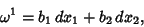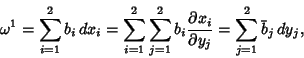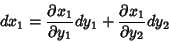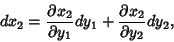## Differential k-Form

A differential-form is a Tensor of Rankwhich is antisymmetric under exchange of any pair of indices. The number of algebraically independent components in-D is, where this is a Binomial Coefficient. In particular, a 1-form (often simply called a differential'') is a quantity(1)

whereandare the components of a Covariant Tensor. Changing variables fromtogives(2)

where(3)

which is the covariant transformation law. 2-forms can be constructed from the Wedge Product of 1-forms. Let(4)(5)

thenis a 2-form denoted. Changing variablestogives(6)(7)

so(8)

Similarly, a 4-form can be constructed from Wedge Products of two 2-forms or four 1-forms(9)

See also Angle Bracket, Bra, Exterior Derivative, Ket, One-Form, Symplectic Form, Wedge Product

References

Weintraub, S. H. Differential Forms: A Complement to Vector Calculus. San Diego, CA: Academic Press, 1996.